maths > vector-algebra

Magnitude of a Vector

what you'll learn...

Overview

Magnitude of a Vector

»  magnitude of $\stackrel{\to }{p}=ai+bj+ck$$\vec{p} = a i + b j + c k$ is $|\stackrel{\to }{p}|=\sqrt{{a}^{2}+{b}^{2}+{c}^{2}}$$| \vec{p} | = \sqrt{{a}^{2} + {b}^{2} + {c}^{2}}$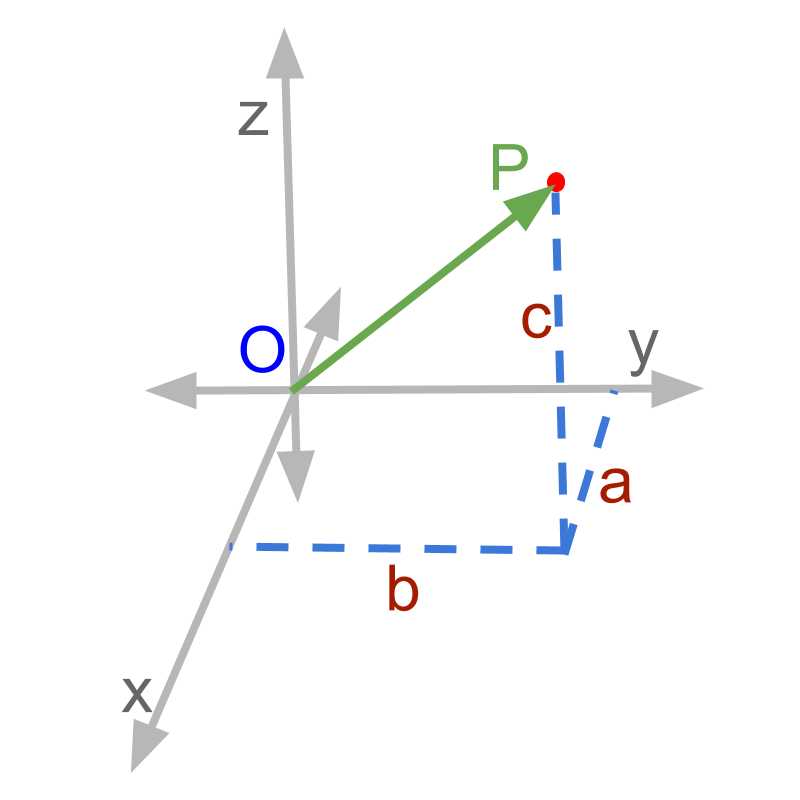→  length of $\overline{OP}$$\overline{O P}$ is the magnitude

length of vector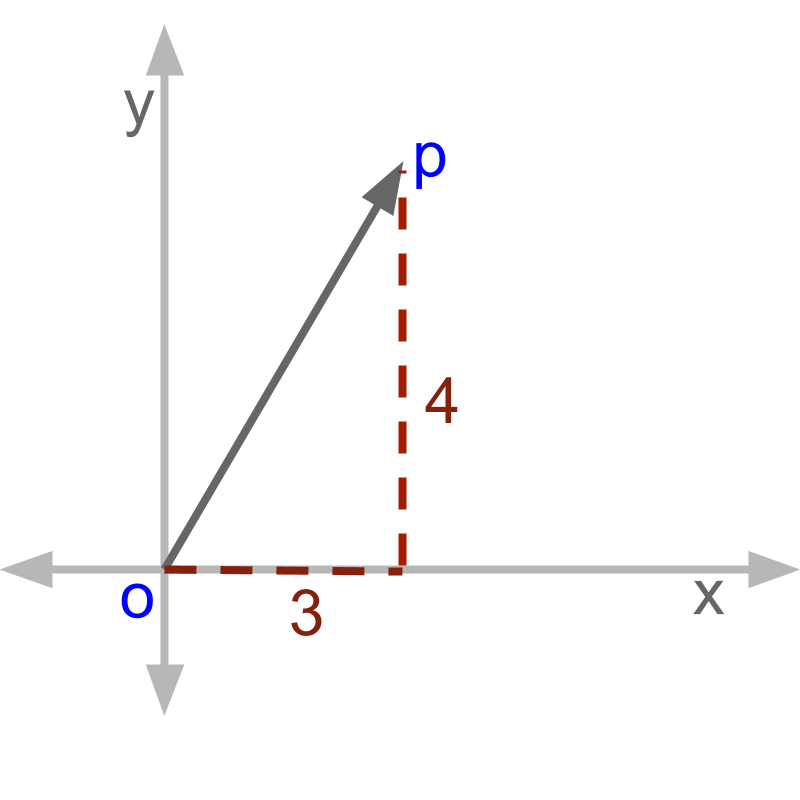The length of the $\stackrel{\to }{OP}$$\vec{O P}$ in the figure is '$\sqrt{{3}^{2}+{4}^{2}}$$\sqrt{{3}^{2} + {4}^{2}}$', which is computed using the formula to find hypotenuse of right angled triangles (Pythagoras theorem).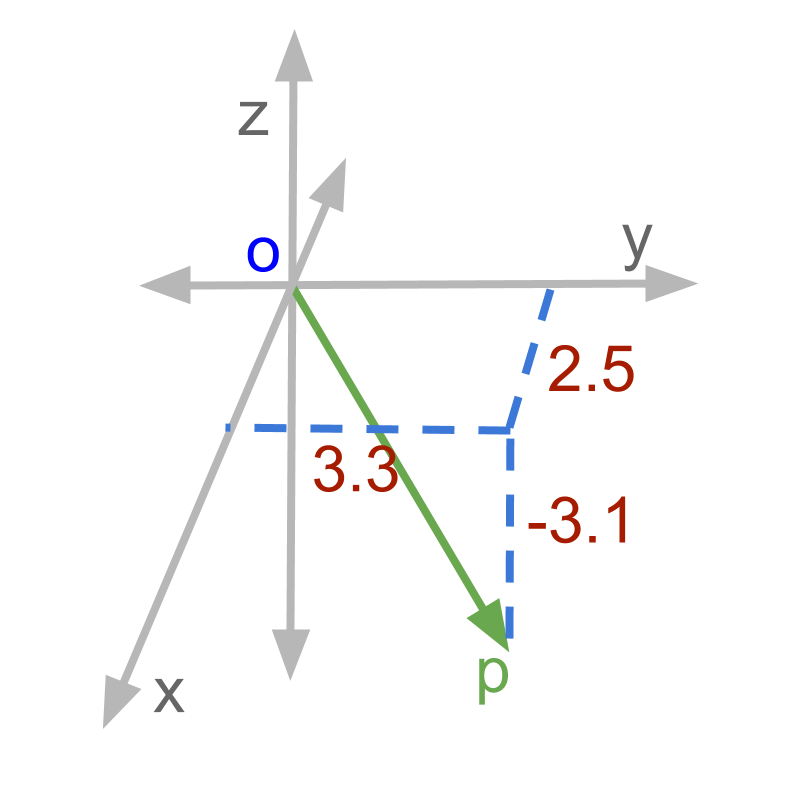The length of the $\stackrel{\to }{OP}$$\vec{O P}$ in the figure is '$\sqrt{{3.3}^{2}+{2.5}^{2}+{\left(-3.1\right)}^{2}}\prime$$\sqrt{{3.3}^{2} + {2.5}^{2} + {\left(- 3.1\right)}^{2}} '$.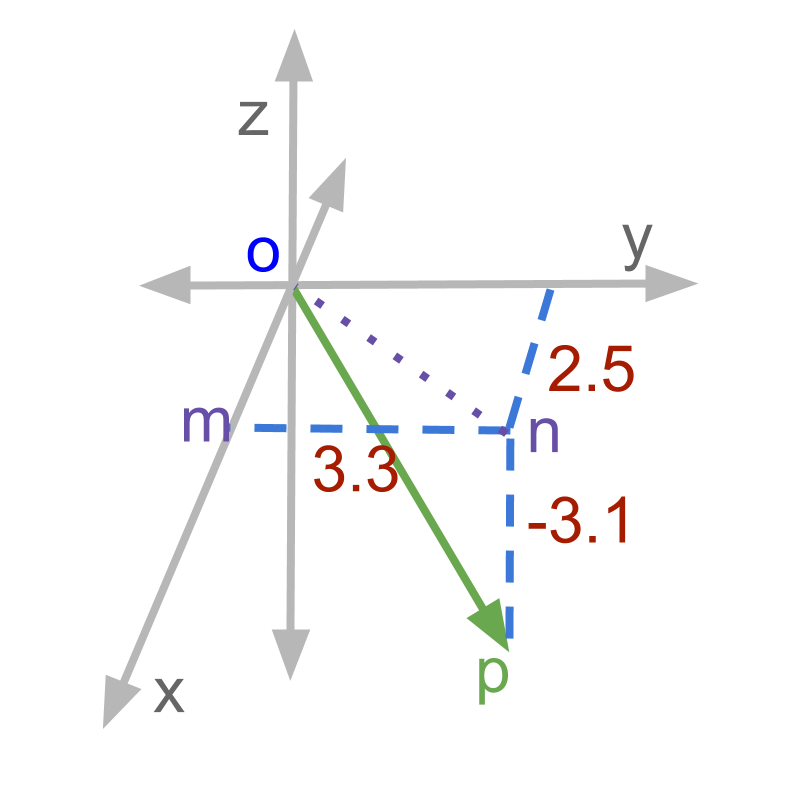The steps to find length of the vector $\stackrel{\to }{O}P$$\vec{O} P$ are

•   length of $\overline{ON}$$\overline{O N}$ is calculated using $\overline{OM}$$\overline{O M}$ and $\overline{MN}$$\overline{M N}$ as sides of a right angled triangle $OMN$$O M N$

•   length of $\overline{OP}$$\overline{O P}$ is calculated using $\overline{ON}$$\overline{O N}$ and $\overline{NP}$$\overline{N P}$ as sides of a right angled triangle $ONP$$O N P$

This uses Pythagoras Theorem in two steps.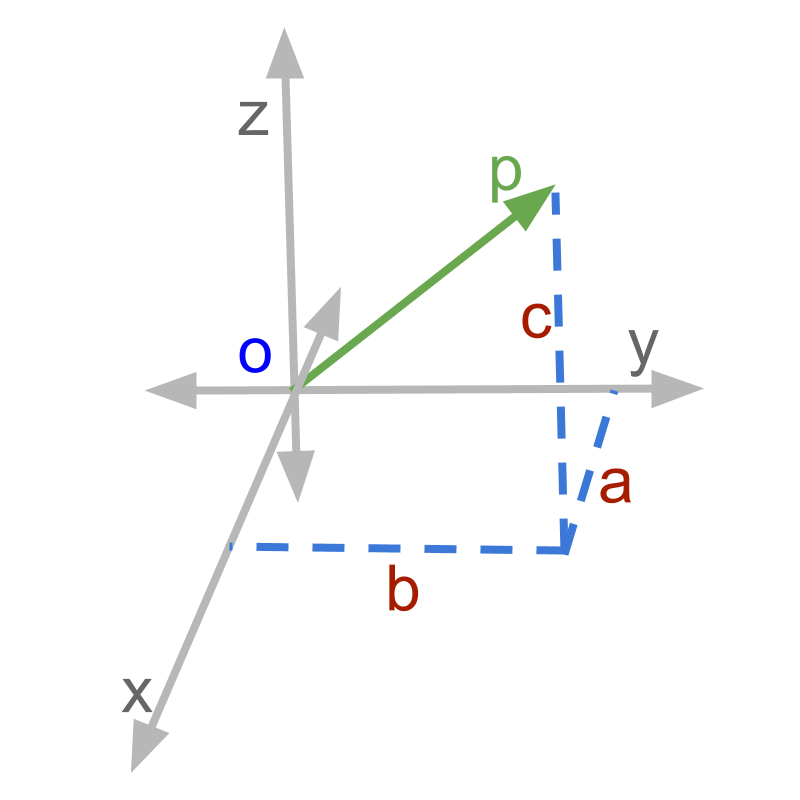A vector is defined as a quantity with magnitude and direction. If the direction information in removed, the magnitude of a vector is obtained. In this example, length of $\overline{OP}$$\overline{O P}$ is the magnitude of the vector. The magnitude of a vector $ai+bj+ck$$a i + b j + c k$ is given by $\sqrt{{a}^{2}+{b}^{2}+{c}^{2}}$$\sqrt{{a}^{2} + {b}^{2} + {c}^{2}}$

magnitude

Magnitude of a vector is the 'amount' of the quantity without the direction information.

Magnitude of a Vector: For a vector $\stackrel{\to }{p}=ai+bj+ck$$\vec{p} = a i + b j + c k$ the magnitude is
$|\stackrel{\to }{p}|=\sqrt{{a}^{2}+{b}^{2}+{c}^{2}}$$| \vec{p} | = \sqrt{{a}^{2} + {b}^{2} + {c}^{2}}$

The word 'magnitude' means size of something.

A point P is given by the vector $2i+4j-2k$$2 i + 4 j - 2 k$. What is the distance of the point from the origin? The answer is $\sqrt{{2}^{2}+{4}^{2}+{\left(-2\right)}^{2}}$$\sqrt{{2}^{2} + {4}^{2} + {\left(- 2\right)}^{2}}$

summary

Magnitude of a Vector: For a vector $\stackrel{\to }{p}=ai+bj+ck$$\vec{p} = a i + b j + c k$ the magnitude is
$|\stackrel{\to }{p}|=\sqrt{{a}^{2}+{b}^{2}+{c}^{2}}$$| \vec{p} | = \sqrt{{a}^{2} + {b}^{2} + {c}^{2}}$

Magnitude is the 'amount' of the quantity without the direction information.

Outline